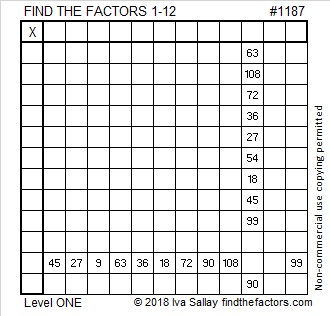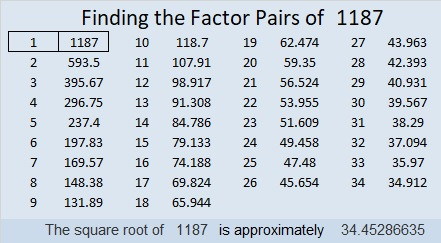# 1187 and Level 1

What is the biggest number that can divide all the clues in today’s puzzle without leaving a remainder? If you can answer that question, then you also know the greatest common factor of all those clues. It really is that simple. You can solve this puzzle!Print the puzzles or type the solution in this excel file: 12 factors 1187-1198

Now I’ll share some information about the number 1187:

• 1187 is a prime number.
• Prime factorization: 1187 is prime.
• The exponent of prime number 1187 is 1. Adding 1 to that exponent we get (1 + 1) = 2. Therefore 1187 has exactly 2 factors.
• Factors of 1187: 1, 1187
• Factor pairs: 1187 = 1 × 1187
• 1187 has no square factors that allow its square root to be simplified. √1187 ≈ 34.45287

How do we know that 1187 is a prime number? If 1187 were not a prime number, then it would be divisible by at least one prime number less than or equal to √1187 ≈ 34.5. Since 1187 cannot be divided evenly by 2, 3, 5, 7, 11, 13, 17, 19, 23, 29 or 31, we know that 1187 is a prime number.1187 is the sum of the nineteen prime numbers from 23 to 103.
It is also the sum of three consecutive primes:
389 + 397 + 401 = 1187

This site uses Akismet to reduce spam. Learn how your comment data is processed.